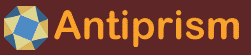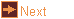# EXTRA: string_art - string figures

 Usage    |    Examples    |    Notes

## Usage

```

Usage: string_art [options]

Create a string art in OFF fomat. Each pin arrangement shape is
modified by any immediately following transformation options.

Options
-h,--help this help message (run 'off_util -H help' for general help)
--version version information
-c <num>  a unit circle arrangement of num pins
-l <num>  a 2 unit line arrangement of num pins
-p <crds> line end points coordinates, six numbers separated by commas
-T <tran> translate, three numbers separated by commas which are
used as the x, y and z displacements
-R <rot>  rotate about an axis, three, four or six numbers separated by
commas. If three numbers these are angles (degrees) to rotate
about the x, y and z axes. If four numbers, the first three
are a direction vector for the axis, the last number is the
angle (degrees) to rotate. If six numbers, these are two
vectors and rotate to carry the first to the second
-M <norm> reflect in a plane, three numbers separated by commas which
give a vector normal to the plane of reflection.
-S <scal> scale, one, three or four numbers separated by commas. If one
number then scale by this factor in all directions. If three
numbers these are the factors to scale along the x, y and
z axes. If four numbers, the first three are a direction
vector for the scaling, the last number is the factor to scale
-I        invert
-o <file> write output to file (default: write to standard output)

```

## Examples

Nephroid
```string_art -c 180 -c 60 -o nephroid.off
```

Astroid
```string_art -c 120 -S 1,0,0 -c 120 -S 0,1,0 -o astroid.off
```

## Notes

The elements can be placed anywhere in space. Attractive figures can be made by running strings between the edges of a polyhedron. The poly_kscope program can help with this. Example stella octangula
```string_art -l 20 -T 1,0,1.414 -R 0,0,45 -l 20 -T -1,0,-1.414 -R 0,0,-45 | poly_kscope -s O > st_oct.off
```